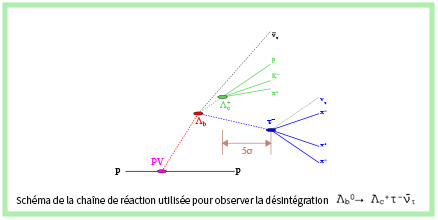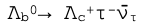LHCb presents at the Lepton Photon 2021 conference a new test of lepton universality, one of the basic principles of the Standard Model of particle physics. This principle states that the Standard Model treats the three charged leptons (electrons, muons and taus) identically, except for differences due to their different masses. The new measurement constitutes the first observation of the semileptonic decay of the baryonand compares it to the corresponding decay in which the τ lepton is replaced by the muon using the ratio of the probabilities of decays R(Λc+).

The study of decaysis particularly challenging. The Λb0 baryon decays at a distance of about a cm from the proton-proton collision point. The decay of the τ lepton produces these three pions along with a neutrino. This 3-pion decay, only exploited by LHCb up to now, has two advantages: the precise measurement of lepton decay point which enables a very strong background rejection, and the exploitation of the dynamics of the 3 pion system which makes it possible to distinguish the decays of taus from those originating from charmed mesons. This technique was developed by the LHCb team of IJCLab who carried out research in 2017 in the similar measurement of R(D*). The statistical significance of the signal is very large (about six standard deviations), so this study is the first observation of this reaction. The observed value of R(Λc+) is in good agreement but a little lower than the prediction of the Standard Model. Some models of new physics, compatible with similar decays already observed and studied by the R(D*) and R(J/ψ) reports predict values of R(Λc+) very different from the Standard Model. The measurement of LHCb will certainly trigger discussions within the theoretical physics community.

To see the paper.